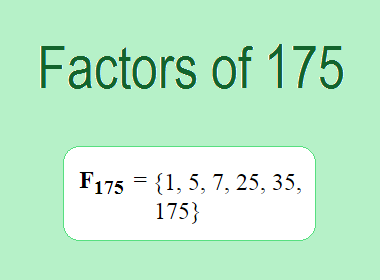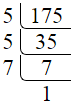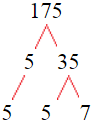# Factors of 175The factors of 175 are 1, 5, 7, 25, 35, and 175 i.e. F175 = {1, 5, 7, 25, 35, 175}. The factors of 175 are all the numbers that can divide 175 without leaving a remainder.

We can check if these numbers are factors of 175 by dividing 175 by each of them. If the result is a whole number, then the number is a factor of 175. Let's do this for each of the numbers listed above:

·        1 is a factor of 175 because 175 divided by 1 is 175.

·        5 is a factor of 175 because 175 divided by 5 is 35.

·        7 is a factor of 175 because 175 divided by 7 is 25.

·        25 is a factor of 175 because 175 divided by 25 is 7.

·        35 is a factor of 175 because 175 divided by 35 is 5.

·        175 is a factor of 175 because 175 divided by 175 is 1.

## How to Find Factors of 175?

1 and the number itself are the factors of every number. So, 1 and 175 are two factors of 175. To find the other factors of 175, we can start by dividing 175 by the numbers between 1 and 175. If we divide 175 by 2, we get a remainder of 1. Therefore, 2 is not a factor of 175. If we divide 175 by 3, we get a remainder of 1. Therefore, 3 is not a factor of 175.

Next, we can check if 4 is a factor of 175. If we divide 175 by 4, we get a remainder of 3. Therefore, 4 is not a factor of 175. If we divide 175 by 5, we get a remainder of 0. Therefore, 5 is a factor of 175. We can continue this process for all the possible factors of 175.

Through this process, we can find that the factors of 175 are 1, 5, 7, 25, 35, and 175. These are the only numbers that can divide 175 without leaving a remainder.

********************

********************

********************

## Properties of the Factors of 175

The factors of 175 have some interesting properties. One of the properties is that the sum of the factors of 175 is equal to 248. We can see this by adding all the factors of 175 together:

1 + 5 + 7 + 25 + 35 + 175 = 248

Another property of the factors of 175 is that they are all odd numbers. This is because 175 is an odd number, and any even number cannot divide an odd number without leaving a remainder.

Another property of the factors of 175 is that the prime factors of 175 are 5 and 7 only.

## Applications of the Factors of 175

The factors of 175 have several applications in mathematics. One of the applications is in finding the highest common factor (HCF) of two or more numbers. The HCF is the largest factor that two or more numbers have in common. For example, to find the HCF of 175 and 28, we need to find the factors of both numbers and identify the largest factor they have in common. The factors of 175 are 1, 5, 7, 25, 35, and 175. The factors of 28 are 1, 2, 4, 7, 14, and 28. The largest factor that they have in common is 7. Therefore, the HCF of 175 and 28 is 7.

Another application of the factors of 175 is in prime factorization. Prime factorization is the process of expressing a number as the product of its prime factors. The prime factors of 175 are 5 and 7 since these are the only prime numbers that can divide 175 without leaving a remainder. Therefore, we can express 175 as:

175 = 5 × 5 × 7

We can do prime factorization by division and factor tree method also. Here is the prime factorization of 175 by division method,175 = 5 × 5 × 7

Here is the prime factorization of 175 by the factor tree method,175 = 5 × 5 × 7

## Conclusion

The factors of 175 are the numbers that can divide 175 without leaving a remainder. The factors of 175 are 1, 5, 7, 25, 35, and 175. The factors of 175 have some interesting properties, such as being odd numbers and having a sum of 248. The factors of 175 have several applications in mathematics, such as finding the highest common factor and prime factorization.